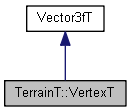TerrainT::VertexT Struct Reference
Inheritance diagram for TerrainT::VertexT:[legend]

## Public Attributes

float e
Error. More...

float rPublic Attributes inherited from Vector3T< float >
float x
The x-component of this vector. More...

float y
The y-component of this vector. More...

float z
The z-component of this vector. More...Public Member Functions inherited from Vector3T< float >
Vector3T ()
The default constructor. It initializes all components to zero. More...

Vector3T (floatx_, floaty_, floatz_)
This constructor initializes the components from x_, y_ and z_ respectively. More...

Vector3T (const C Values[])
This constructor initializes the components from an array of (at least) three Ts. More...

bool IsValid () const
Returns true if the vector is valid, that is, all components are non-NANs. More...

float & operator[] (unsigned int Index)
Component access by index number (0 to 2) rather than by name. More...

const float & operator[] (unsigned int Index) const
Component access by index number (0 to 2) rather than by name. More...

Vector3T< float > AsVectorOfFloat () const
Gets this Vector3T<T> as a Vector3T<float>, so that the cast is explicitly visible in user code. More...

Vector3T< double > AsVectorOfDouble () const
Gets this Vector3T<T> as a Vector3T<double>, so that the cast is explicitly visible in user code. More...

Vector3T< int > AsVectorOfInt () const
Gets this Vector3T<T> as a Vector3T<int>, so that the cast is explicitly visible in user code. More...

float GetLengthSqr () const
Returns the square of the length of this vector. More...

bool IsEqual (const Vector3T< float > &B, const floatEpsilon) const
Returns whether this vector is equal to B within tolerance Epsilon, that is, whether it is geometrically closer to B than Epsilon. More...

Vector3T< float > GetScaled (const floats) const
Returns a copy of this vector scaled by s, that is, the scalar product (Skalarmultiplikation) of this vector and s. More...

Vector3T< float > GetScaled (const Vector3T< float > &S) const
Returns a copy of this vector non-uniformely scaled by S. More...

Vector3T< float > GetRotX (const floatAngle) const
Returns a copy of this vector rotated around the x-axis by Angle degrees. More...

Vector3T< float > GetRotY (const floatAngle) const
Returns a copy of this vector rotated around the y-axis by Angle degrees. More...

Vector3T< float > GetRotZ (const floatAngle) const
Returns a copy of this vector rotated around the z-axis by Angle degrees. More...

void CreateOrthoVectors (Vector3T< float > &Left, Vector3T< float > &Down) const
Returns two vectors that are orthogonal to this vector and to each other. More...

bool operator== (const Vector3T< float > &B) const
Returns whether this vector and B are truly (bit-wise) identical. More...

bool operator!= (const Vector3T< float > &B) const
Returns whether this vector and B are not equal (bit-wise). More...

Vector3T< float > operator+ (const Vector3T< float > &B) const
Returns the sum of this Vector3T<T> and B. More...

Vector3T< float > operator- (const Vector3T< float > &B) const
Returns the difference between this Vector3T<T> and B. More...

Vector3T< float > operator- () const
The unary minus operator. B=-A is quasi identical with B=A.GetScaled(-1). More...

Vector3T< float > operator* (const floats) const
Returns a copy of this vector scaled by s, that is, the scalar product (Skalarmultiplikation) of this vector and s. More...

Vector3T< float > operator/ (const floats) const
Returns a copy of this vector divided by s, that is, the scalar product (Skalarmultiplikation) of this vector and 1/s. More...

float dot (const Vector3T< float > &B) const
Returns the dot product (Skalarprodukt) of this vector and B. More...

Vector3T< float > cross (const Vector3T< float > &B) const
Returns the cross product (Vektorprodukt) of this vector and B. More...

Vector3T< float > & operator+= (const Vector3T< float > &B)
Adds B to this vector. More...

Vector3T< float > & operator-= (const Vector3T< float > &B)
Subtracts B from this vector. More...

Vector3T< float > & operator*= (const floats)
Scales this vector by s. More...

Vector3T< float > & operator/= (const floats)
Divides this vector by s. Assumes that s is not 0. More...

## Member Data Documentation

 float TerrainT::VertexT::e

Error.

 float TerrainT::VertexT::r